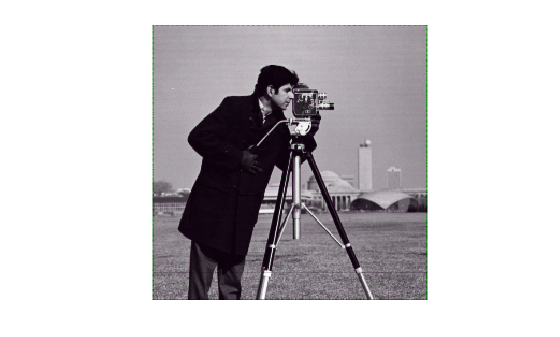Main Content

# imregcorr

Estimate geometric transformation that aligns two 2-D images using phase correlation

## Syntax

``tform = imregcorr(moving,fixed)``
``tform = imregcorr(moving,Rmoving,fixed,Rfixed)``
``tform = imregcorr(___,transformType)``
``tform = imregcorr(___,'Window',window)``
``[tform,peakcorr] = imregcorr(___)``

## Description

example

````tform = imregcorr(moving,fixed)` estimates the geometric transformation that aligns an image, `moving`, with a reference image, `fixed`. The function returns a geometric transformation object, `tform`, that maps pixels in `moving` to pixels in `fixed`. ```
````tform = imregcorr(moving,Rmoving,fixed,Rfixed)` estimates the geometric transformation that aligns an image, `moving`, with a reference image, `fixed`. `Rmoving` and `Rfixed` are spatial referencing objects that contain spatial information about the `moving` and `fixed` images, respectively. The transformation object returned, `tform`, defines the point mapping in the world coordinate system.```
````tform = imregcorr(___,transformType)` also specifies the type of transformation, `transformType`.```
````tform = imregcorr(___,'Window',window)` also specifies whether to perform windowing in the frequency domain. To increase the stability of registration results, specify `window` as `true`. However, if the common features in your images are oriented along the edges, then setting `window` to `false` can sometimes provide superior registration results.```
````[tform,peakcorr] = imregcorr(___)` also returns the peak correlation, `peakcorr`, of the phase difference between the two images.```

## Examples

collapse all

Read a reference image into the workspace.

`fixed = imread('cameraman.tif');`

Create a synthetic moving image by scaling and rotating the fixed image.

```theta = 20; S = 2.3; tform = affine2d([S.*cosd(theta) -S.*sind(theta) 0; ... S.*sind(theta) S.*cosd(theta) 0; ... 0 0 1]); moving = imwarp(fixed,tform); moving = moving + uint8(10*rand(size(moving)));```

Display the fixed and the moving image alongside each other.

`imshowpair(fixed,moving,'montage')`Estimate the transformation needed to align the images using `imregcorr`.

`tformEstimate = imregcorr(moving,fixed);`

Apply estimated geometric transform to the moving image. This example uses the `'OutputView'` parameter to obtain a registered image the same size and with the same world limits as the reference image.

```Rfixed = imref2d(size(fixed)); movingReg = imwarp(moving,tformEstimate,'OutputView',Rfixed);```

View the original image and the registered image side-by-side to check the registration. Then view the registered image overlaid on the original using the `'falsecolor'` option to highlight any areas where the images differ.

`imshowpair(fixed,movingReg,'montage')``imshowpair(fixed,movingReg,'falsecolor');`## Input Arguments

collapse all

Image to be registered, specified as a grayscale, binary, or RGB image. If you specify an RGB image, `imregcorr` converts it to a grayscale image using `rgb2gray` before processing.

Note

The aspect ratio of `moving` affects the output transform `tform`. For best results, use a square image.

Data Types: `single` | `double` | `int8` | `int16` | `int32` | `uint8` | `uint16` | `uint32` | `logical`

Reference image in the target orientation, specified as a grayscale, binary, or RGB image. If you specify an RGB image, `imregcorr` converts it to a grayscale image using `rgb2gray` before processing.

Note

The aspect ratio of `fixed` affects the output transform `tform`. For best results, use a square image.

Data Types: `single` | `double` | `int8` | `int16` | `int32` | `uint8` | `uint16` | `uint32` | `logical`

Type of transformation to estimate, specified as one of the following values.

ValueDescription
`'translation'`Translation
`'rigid'`Translation and rotation
`'similarity'`

Translation, rotation, and scaling

When using the `'similarity'` option, the phase correlation algorithm is only scale invariant within some range of scale difference between the fixed and moving images. `imregcorr` limits the search space to scale differences within the range [1/4, 4]. `imregcorr` does not detect scale differences less than 1/4 or greater than 4.

Data Types: `char` | `string`

Spatial referencing information associated with the image to be registered, specified as an `imref2d` object.

Spatial referencing information associated with the reference (fixed) image, specified as an `imref2d` object.

Use windowing to suppress spectral leakage effects in the frequency domain, specified as a numeric or logical `1` (`true`) or `0` (`false`). When `true`, the `imregcorr` function performs windowing using a Blackman filter.

## Output Arguments

collapse all

Geometric transformation, returned as an `affine2d` object.

Peak correlation value of the phase difference between the two images, returned as a numeric scalar.

## Tips

• If your image is of type `double`, you can achieve performance improvements by casting the image to `single` with `im2single` before registration. Input images of type `double` cause the algorithm to compute FFTs in `double`.

 Reddy, B. S. and Chatterji, B. N., An FFT-Based Technique for Translation, Rotation, and Scale-Invariant Image Registration, IEEE Transactions on Image Processing, Vol. 5, No. 8, August 1996

Download ebook